Solving difficult equations

Chapter 2 Class 8 Linear Equations in One Variable
Serial order wise

To do this question, first check Two digit number and its reverse .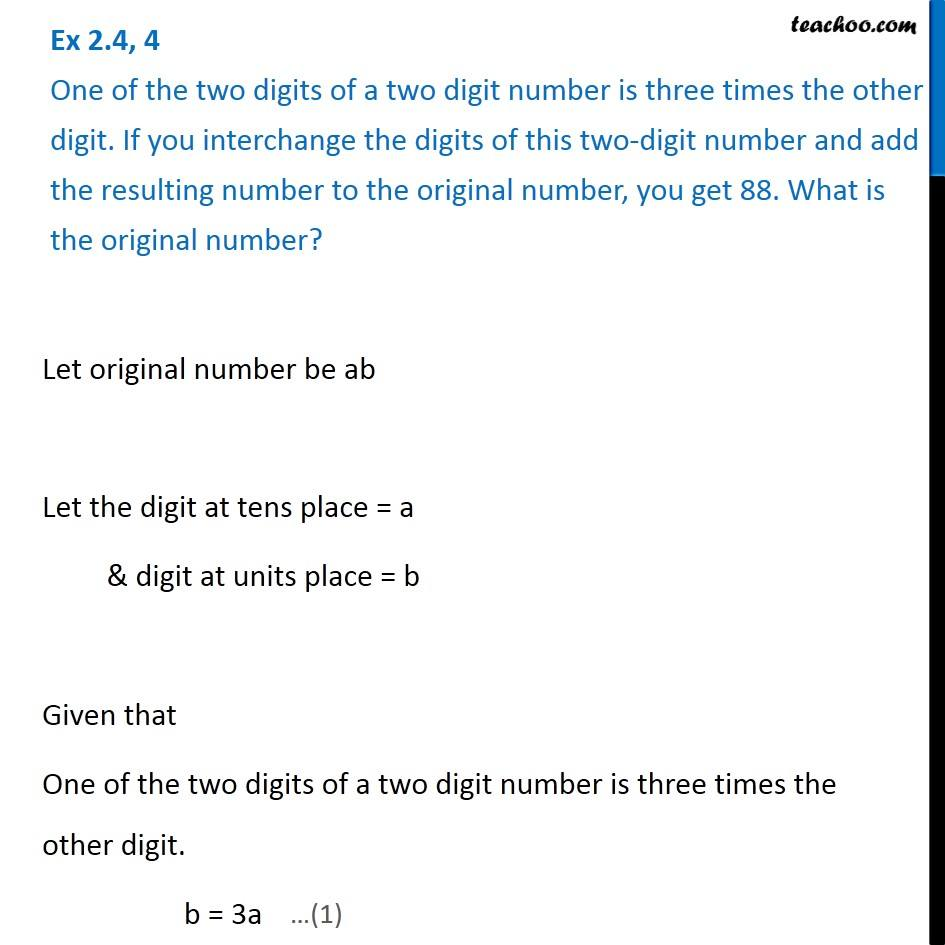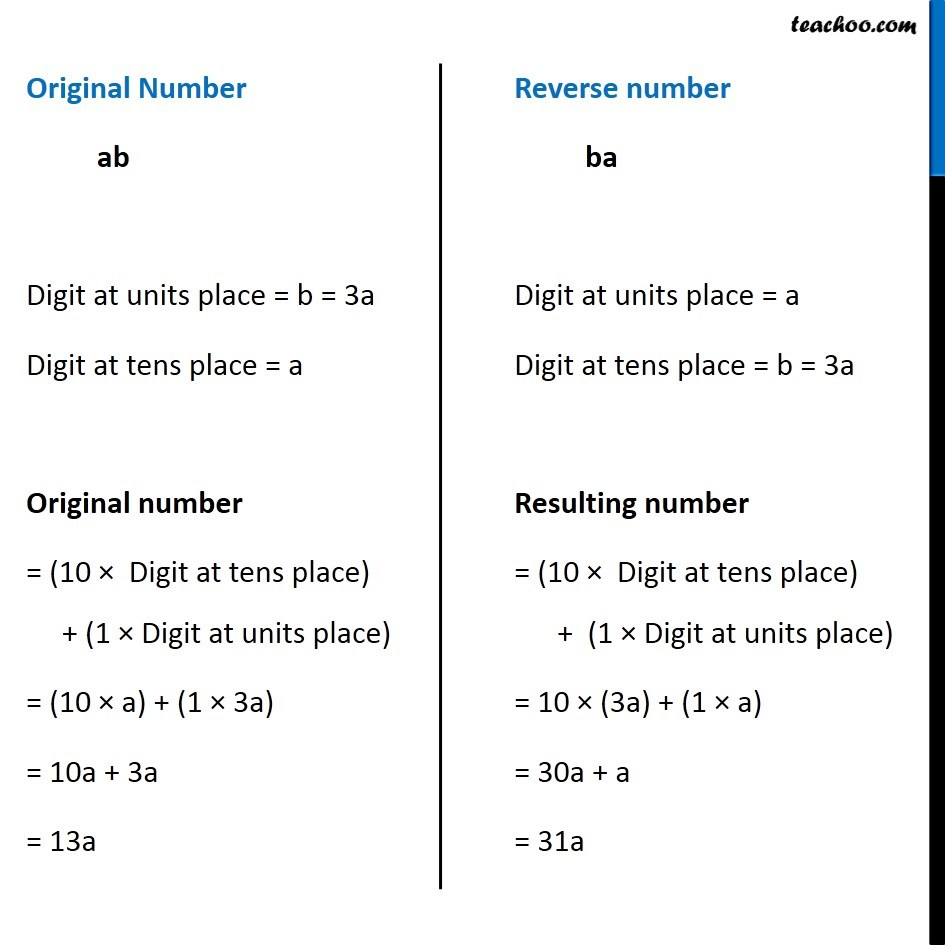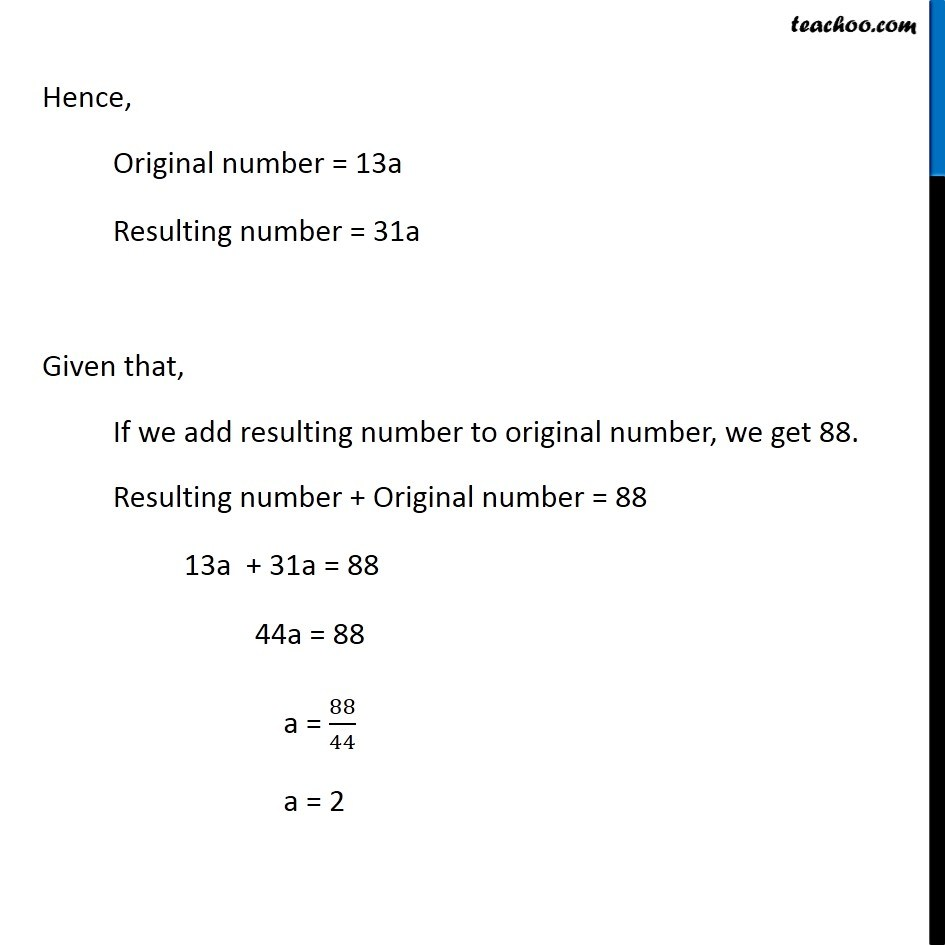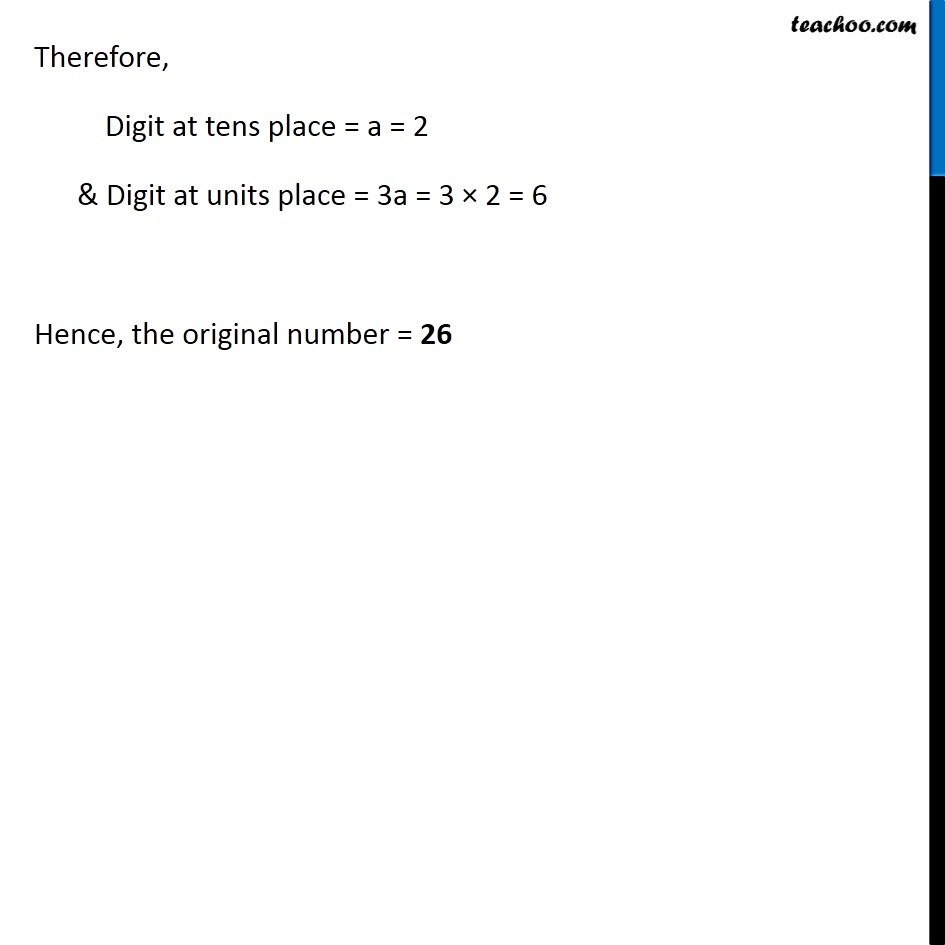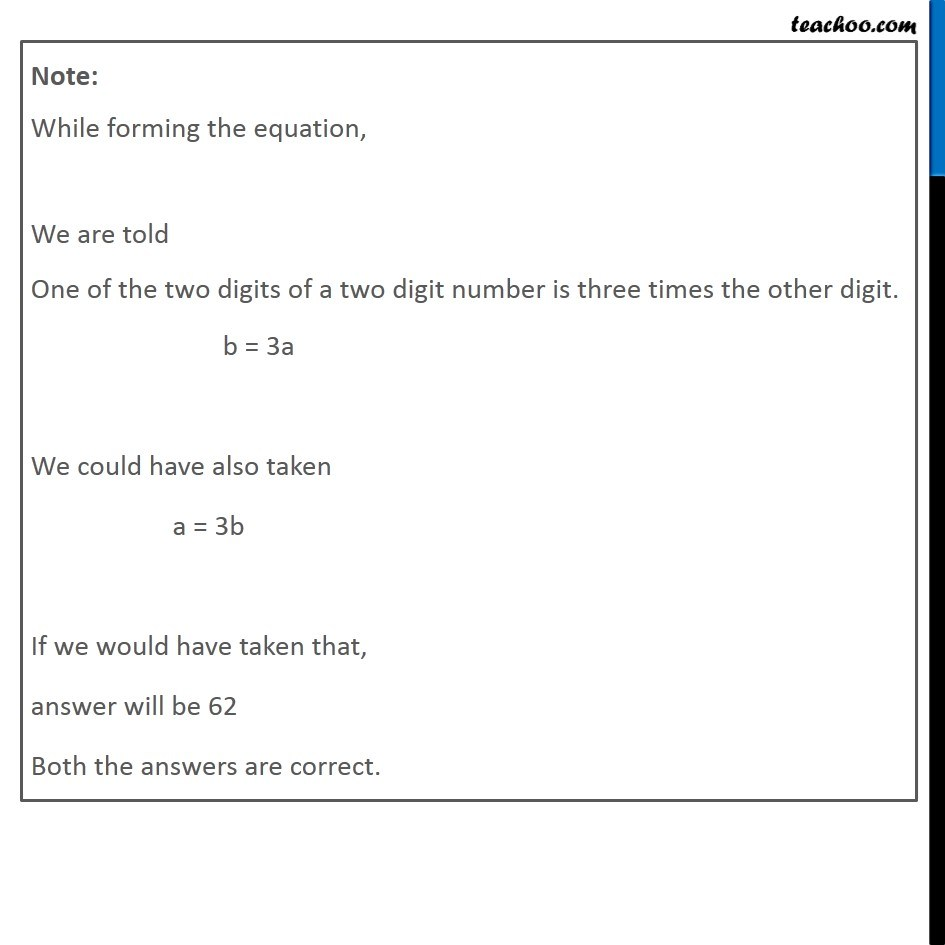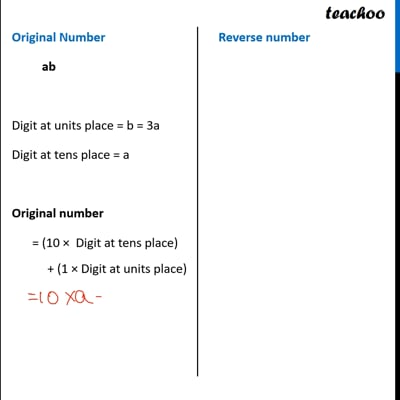This video is only available for Teachoo black users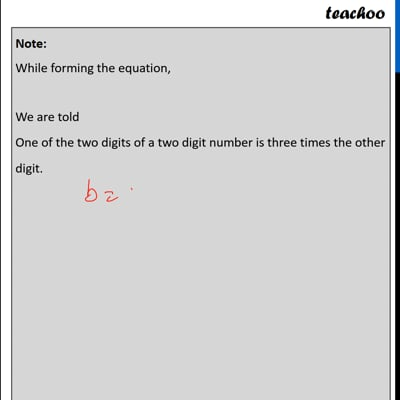This video is only available for Teachoo black users

Learn in your speed, with individual attention - Teachoo Maths 1-on-1 Class

### Transcript

Question 4 One of the two digits of a two digit number is three times the other digit. If you interchange the digits of this two-digit number and add the resulting number to the original number, you get 88. What is the original number?Let original number be ab Let the digit at tens place = a & digit at units place = b Given that One of the two digits of a two digit number is three times the other digit. b = 3a Original Number ab Digit at units place = b = 3a Digit at tens place = a Original number = (10 × Digit at tens place) + (1 × Digit at units place) = (10 × a) + (1 × 3a) = 10a + 3a = 13a Reverse number ba Digit at units place = a Digit at tens place = b = 3a Resulting number = (10 × Digit at tens place) + (1 × Digit at units place) = 10 × (3a) + (1 × a) = 30a + a = 31a Hence, Original number = 13a Resulting number = 31a Given that, If we add resulting number to original number, we get 88. Resulting number + Original number = 88 13a + 31a = 88 44a = 88 a = 88/44 a = 2 Therefore, Digit at tens place = a = 2 & Digit at units place = 3a = 3 × 2 = 6 Hence, the original number = 26 Note: While forming the equation, We are told One of the two digits of a two digit number is three times the other digit. b = 3a We could have also taken a = 3b If we would have taken that, answer will be 62 Both the answers are correct.